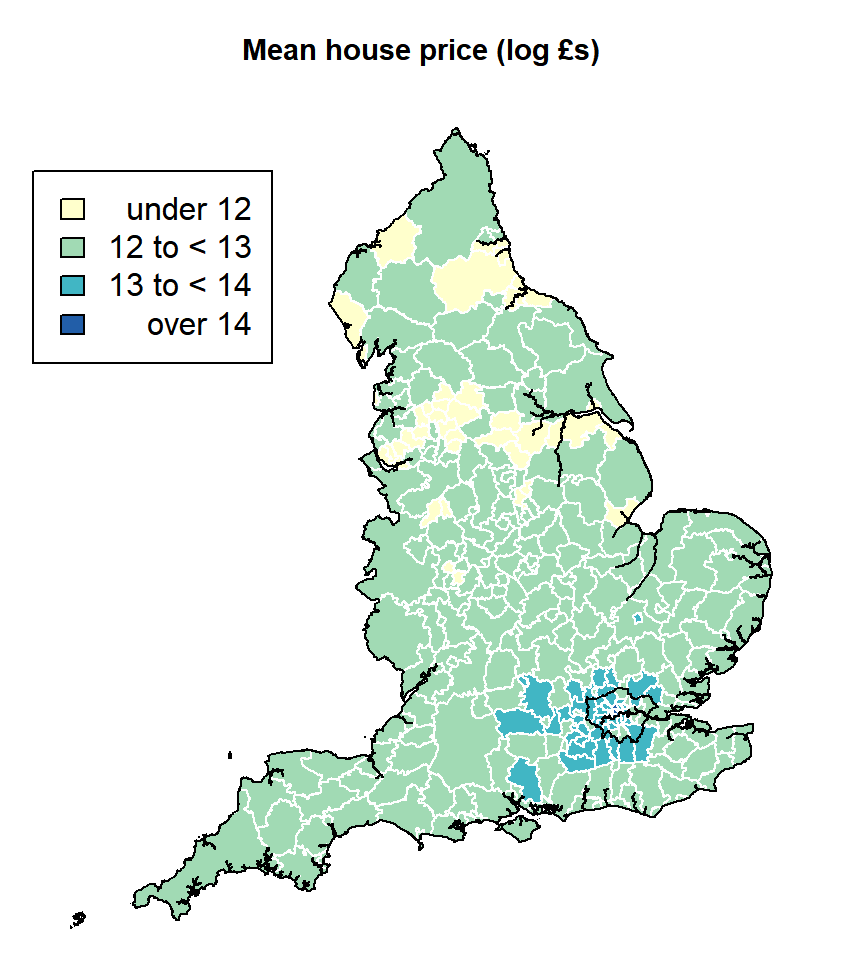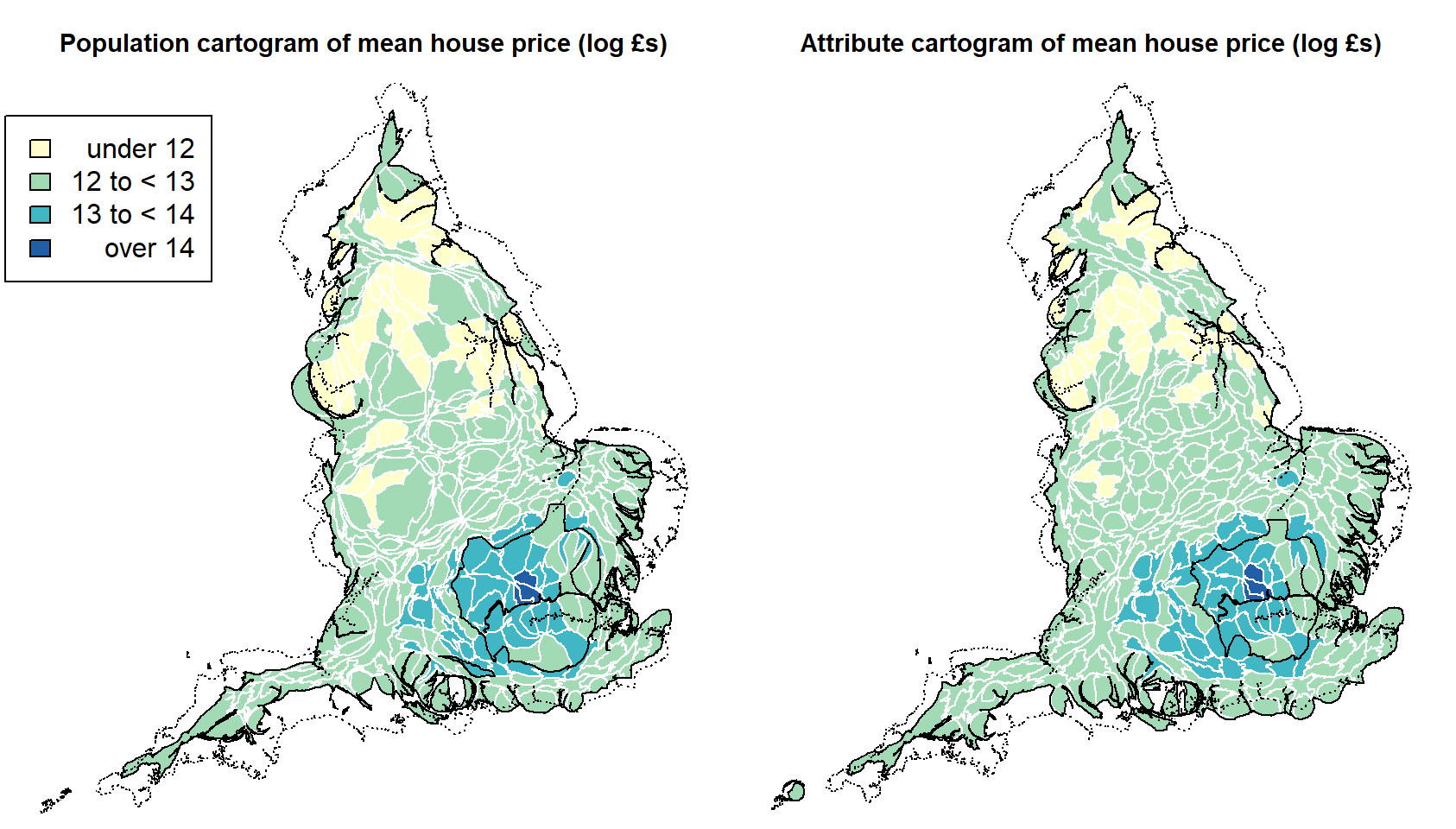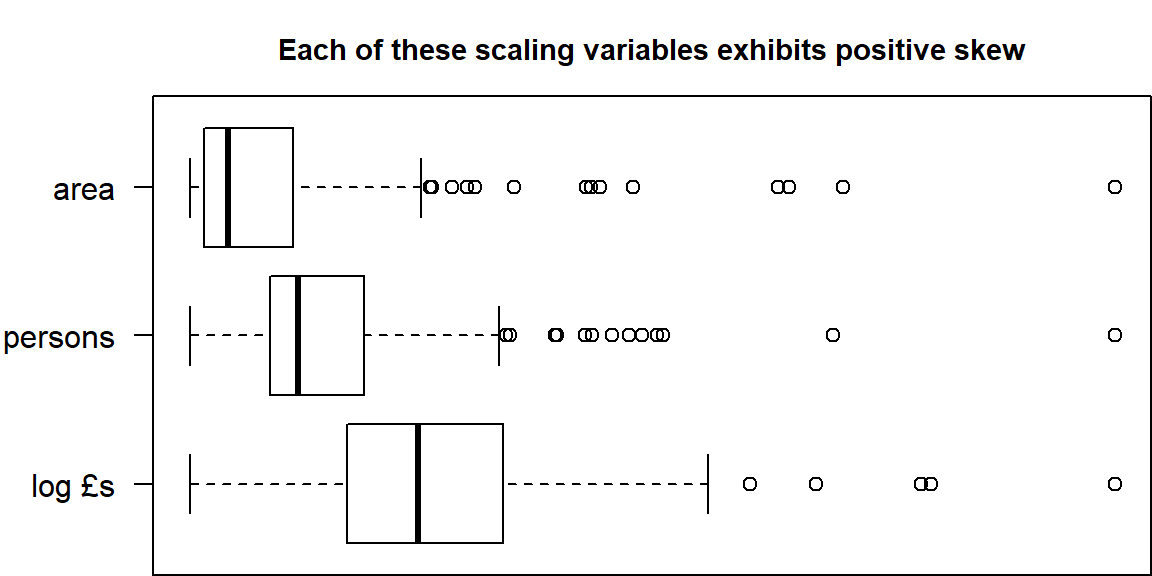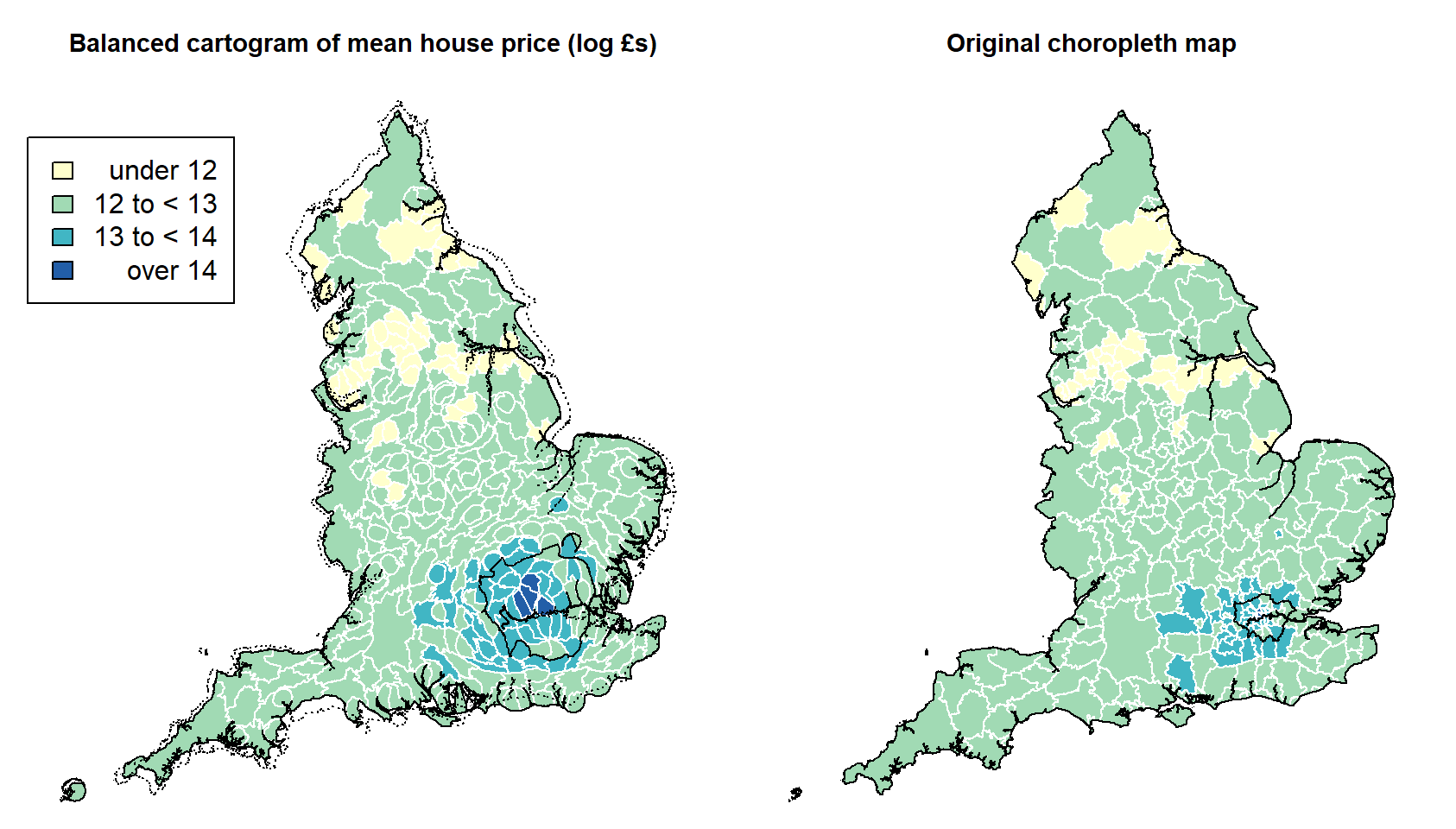## Introduction

This tutorial explores the use of hexograms to produce better maps of area based data and population distributions. Hexograms are a cross between hexagonal binning, tile maps and cartograms that aim to redress the problem of ‘invisibility’ prevalent in conventional maps and also the problem of distortion caused by cartograms. Examples of hexograms and the functions to produce them in R are presented. In principle those functions will work with other data (specifically, spatial polygons and spatial polygon data frames). However, it is a proof of concept not fully developed code and it’s not at all optimised for speed.

### To get started

# Yours will differ from what is written below
setwd("C:/Users/profr/Downloads")
##### Install the required libraries
reqd <- c("cartogram", "sp", "fMultivar", "RANN","rgdal","rgeos","GISTools",
"RCurl")
pkgs <- rownames(installed.packages())
need <- reqd[!reqd %in% pkgs]
if(length(need)) install.packages(need)
download.file("https://github.com/profrichharris/Rhexogram/blob/master/las.zip?raw=true", "las.zip", mode="wb")
unzip("las.zip")
map <- rgdal::readOGR("las.shp", "las")

## Illustrating the problem

A perennial problem when mapping neighbourhood data is the variable size of the areas. Typically this leaves the map dominated by the largest areas - which often contain the fewest people - whereas the attributes of the smallest areas become too small to discern. An example is shown below, which is a choropleth map of the log of the average house price of local authorities in England in 2016. The highest prices are in London - the boundary of which is shown - but where in the capital is most expensive and the spatial variation within it are not clear.

require(GISTools)
z <- log(map$MeanPrice) shades <- auto.shading(z, cutter = rangeCuts, n = 4, cols = brewer.pal(4, "YlGnBu")) par(mai=c(0,0,0.5,0)) choropleth(map, z, shading = shades, border = "white") outline <- rgeos::gBuffer(map) plot(outline, add = T) plot(rgeos::gBuffer(map[map$RGN == "London",]), add=T)
choro.legend(52473, 622458, shades, between = "to <")
title("Mean house price (log £s)", cex.main = 0.9)A ‘solution’ has been to use cartograms that resize the areas in accordance with their population size or, alternatively, in proportion to the attribute of interest, presently the log of the average house price. Both approaches are shown below, and were produced using the cartogram package for R. The concern - which is not a criticism of the package but a comment on cartograms more generally - is that these trade invisibility for distortion: London is now much larger but it comes at the price of geographic distortion that renders parts of the rest of the map illegible. If the purpose of the map is to convey location as well as measurement then neither the choropleth nor the cartograms are especially successful. Both suffer from a problem of misrepresentation.## Balanced cartograms

Harris et al. (2017b) describe the problem of geographic distortion as ‘the curse of the cartogram’. The problem arises not from the method per se but from the choice of scaling variable used to reapportion the areas. Exchanging one positively skewed variable (the area of each local authority), with another positively skewed variable (the house prices) cannot solve the visualisation issue. The problem would, of course, be even worse if the actual prices were used instead of their log.What can? Harris et al. (2017a) suggest addressing the specific problem head on. For the original map, this is that some of the areas are too small to be seen so the obvious solution is to make them bigger. Doing this does not require them to be re-scaled against what is really a fairly arbitrary value like population size; only to ensure that they are big enough to be seen. That requires them to have an area of about 0.02 squared-inches. So, assuming we are producing a map to fit into a graphic window with a height of 5 inches then a process to rescale the map is:

siu <- 0.02 # the smallest interpretable unit
height <- 5
bb <- sp::bbox(map)
width <- (bb[1,2] - bb[1,1]) / (bb[2,2] - bb[2,1]) * height
bbA <- (bb[1,2] - bb[1,1]) * (bb[2,2] - bb[2, 1])
mapA <- rgeos::gArea(map)
minA <- (siu * bbA) / (height * width)
map$scaleby <- rgeos::gArea(map, byid = TRUE) map$scaleby[map$scaleby < minA] <- minA # The following will take a little while to run balcarto <- cartogram::cartogram(map, "scaleby", maxSizeError = 1.1, prepare = "none") This leads to what Harris et al. describe as a balanced cartogram because it better balances invisibility and distortion. par(mai=c(0,0,0.5,0)) par(mfrow=c(1,2)) choropleth(balcarto, z, shading = shades, border = "white") plot(rgeos::gBuffer(balcarto), add=T) plot(rgeos::gBuffer(balcarto[popcarto$RGN == "London",]), add=T)
plot(outline, lty = "dotted", add = T)
choro.legend(52473, 622458, shades, between = "to <")
title("Balanced cartogram of mean house price (log £s)", cex.main = 0.9)
title("Original choropleth map", cex.main = 0.9)If an error is defined as the percentage amount by which the original map and the cartogram do not overlap, then the gain from the balanced cartogram is clear: for the population cartogram the error is 13.9 per cent, for the attribute based cartogram it is 12 per cent and for the balanced cartogram it is halved to 6.2. More precisely, the error may be defined as,

$\epsilon = 100-50A_{{x}\cap{y}}\left(\frac{1}{A_{x}} + \frac{1}{A_{y}}\right)$ where $$A_{x}$$ is the area of the original map, $$A_{y}$$ is the area of the cartogram, and $$A_{{x}\cap{y}}$$ is the area of their geographical intersection.

Another way to consider the amount of distortion is to consider the average displacement of the area centroids from the original map to their new location on the cartogram. This is 26.04km for the population cartogram, 25.18km for the attribute cartogram and 14.19km for the balanced cartogram.

On these two criteria, it is the balanced cartogram that offers the best representation of the house price geography.

## Hexagonal binning

Hexagonal binning has become popular as a method of data visualization and can be used to map the density of point occcurrences, as in this map where the hexagon area encodes the number of Walmart stores that fall into each bin and the colour represents the median age. Although an attractive map, the problems with applying this idea to the house price data are finding room on the map to draw the hexagons for the smallest local authorities, especially in London, and because we are seeking to map area (lattice) data not the density of points.

## Hexograms

The idea behind a hexogram is the same as for the balanced cartogram except that the minimum area is that which allows each area to be represented by as its own hexagon in a process of hexagonal binning, as in the map below.